# OpAmp Oscillator Circuits

• #### Question 1

 Don’t just sit there! Build something!!

Learning to mathematically analyze circuits requires much study and practice. Typically, students practice by working through lots of sample problems and checking their answers against those provided by the textbook or the instructor. While this is good, there is a much better way.

You will learn much more by actually building and analyzing real circuits, letting your test equipment provide the “answers” instead of a book or another person. For successful circuit-building exercises, follow these steps:

1. Carefully measure and record all component values prior to circuit construction.
2. Draw the schematic diagram for the circuit to be analyzed.
3. Carefully build this circuit on a breadboard or other convenient medium.
4. Check the accuracy of the circuit’s construction, following each wire to each connection point, and verifying these elements one-by-one on the diagram.
5. Mathematically analyze the circuit, solving for all voltage and current values.
6. Carefully measure all voltages and currents, to verify the accuracy of your analysis.
7. If there are any substantial errors (greater than a few percent), carefully check your circuit’s construction against the diagram, then carefully re-calculate the values and re-measure.

Avoid using the model 741 op-amp, unless you want to challenge your circuit design skills. There are more versatile op-amp models commonly available for the beginner. I recommend the LM324 for DC and low-frequency AC circuits, and the TL082 for AC projects involving audio or higher frequencies.

As usual, avoid very high and very low resistor values, to avoid measurement errors caused by meter “loading”. I recommend resistor values between 1 kΩ and 100 kΩ.

One way you can save time and reduce the possibility of error is to begin with a very simple circuit and incrementally add components to increase its complexity after each analysis, rather than building a whole new circuit for each practice problem. Another time-saving technique is to re-use the same components in a variety of different circuit configurations. This way, you won’t have to measure any component’s value more than once.

• #### Question 2

Explain what the Barkhausen criterion is for an oscillator circuit. How will the oscillator circuit’s performance be affected if the Barkhausen criterion falls below 1, or goes much above 1?

• #### Question 3

How many degrees of phase shift must the feedback circuit (the square box in this schematic) introduce to the signal in order for this inverting amplifier circuit to oscillate?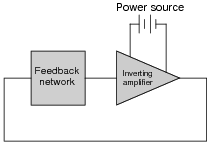• #### Question 4

How many degrees of phase shift must the feedback circuit (the square box in this schematic) introduce to the signal in order for this non-inverting amplifier circuit to oscillate?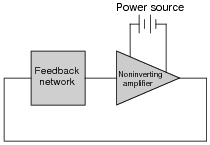• #### Question 5

This is a very common opamp oscillator circuit, technically of the relaxation type:Explain how this circuit works, and what waveforms will be measured at points A and B. Be sure to make reference to RC time constants in your explanation.

• #### Question 6

A variation on the common opamp relaxation oscillator design is this, which gives it variable duty cycle capability:Explain how this circuit works, and which direction the potentiometer wiper must be moved to increase the duty cycle (more time spent with the opamp output saturated at V and less time spent saturated at -V).

• #### Question 7

Dual, or split, power supplies are very useful in opamp circuits because they allow the output voltage to rise above as well as sink below ground potential, for true AC operation. In some applications, though, it may not be practical or affordable to have a dual power supply to power your opamp circuit. In this event, you need to be able to figure out how to adapt your dual-supply circuit to single-supply operation.

A good example of such a challenge is the familiar opamp relaxation oscillator, shown here:First, determine what would happen if we were to simply eliminate the negative portion of the dual power supply and try to run the circuit on a single supply ( V and Ground only):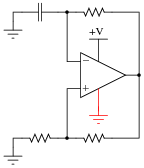Then, modify the schematic so that the circuit will run as well as it did before with the dual supply.

• #### Question 8

Identify what type of oscillator circuit this is, and write an equation describing its operating frequency:• #### Question 9

Explain the purpose of the tank circuit (L1 and C1) in the following oscillator circuit, and write an equation describing its operating frequency:• #### Question 10

This Wien bridge oscillator circuit is very sensitive to changes in the gain. Note how the potentiometer used in this circuit is the “trimmer” variety, adjustable with a screwdriver rather than by a knob or other hand control: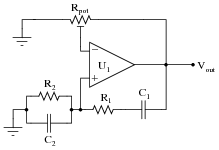The reason for this choice in potentiometers is to make accidental changes in circuit gain less probable. If you build this circuit, you will see that tiny changes in this potentiometer’s setting make a huge difference in the quality of the output sine wave. A little too much gain, and the sine wave becomes noticeably distorted. Too little gain, and the circuit stops oscillating altogether!

Obviously, it is not good to have such sensitivity to minor changes in any practical circuit expected to reliably perform day after day. One solution to this problem is to add a limiting network to the circuit comprised of two diodes and two resistors: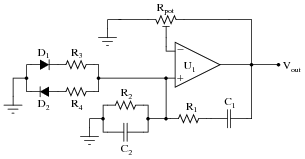With this network in place, the circuit gain may be adjusted well above the threshold for oscillation (Barkhausen criterion) without exhibiting excessive distortion as it would have without the limiting network. Explain why the limiting network makes this possible.

• #### Question 11

This interesting opamp circuit produces true three-phase sinusoidal voltage waveforms, three of them to be exact:With all the resistors and capacitors, you might have guessed this to be a phase-shift type of oscillator circuit, and you would be correct. Here, each parallel RC network provides 60 degrees of lagging phase shift to combine with the 180 degrees of phase shift inherent to the inverting amplifier configurations, yielding 120 degrees of shift per opamp stage.

Derive a formula solving for the operating frequency of this oscillator circuit, knowing that the impedance of each parallel RC network will have a phase angle of -60o. Also, determine where on this circuit you would obtain the three promised sine waves.

• #### Question 12

Predict how the operation of this relaxation oscillator circuit will be affected as a result of the following faults. Consider each fault independently (i.e. one at a time, no multiple faults):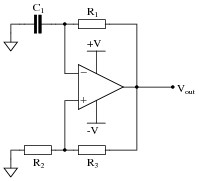Resistor R1 fails open:
Solder bridge (short) across resistor R1:
Capacitor C1 fails shorted:
Solder bridge (short) across resistor R2:
Resistor R3 fails open:

For each of these conditions, explain why the resulting effects will occur.

• #### Question 13

Identify at least two different component faults that would result in a change in duty cycle for this oscillator circuit: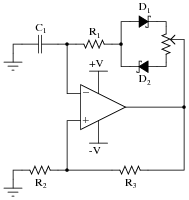• #### Question 14

Suppose this LC oscillator stopped working, and you suspected either the capacitor or the inductor as having failed. How could you check these two components without the use of an LCR meter?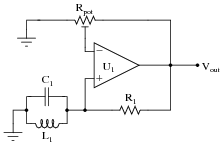• #### Question 15

Most operational amplifiers do not have the ability to swing their output voltages rail-to-rail. Most of those do not swing their output voltages symmetrically. That is, a typical non-rail-to-rail opamp may be able to approach one power supply rail voltage closer than the other; e.g. when powered by a 15/-15 volt split supply, the output saturates positive at 14 volts and saturates negative at -13.5 volts.

What effect do you suppose this non-symmetrical output range will have on a typical relaxation oscillator circuit such as the following, and how might you suggest we fix the problem?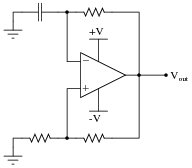• #### Question 16

This resonant LC oscillator circuit is very sensitive to changes in the gain. Note how the potentiometer used in this circuit is the “trimmer” variety, adjustable with a screwdriver rather than by a knob or other hand control: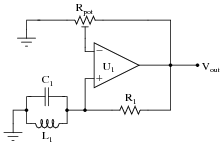The reason for this choice in potentiometers is to make accidental changes in circuit gain less probable. If you build this circuit, you will see that tiny changes in this potentiometer’s setting make a huge difference in the quality of the output sine wave. A little too much gain, and the sine wave becomes noticeably distorted. Too little gain, and the circuit stops oscillating altogether!

Obviously, it is not good to have such sensitivity to minor changes in any practical circuit expected to reliably perform day after day. One solution to this problem is to add a limiting network to the circuit comprised of two diodes and two resistors:With this network in place, the circuit gain may be adjusted well above the threshold for oscillation (Barkhausen criterion) without exhibiting excessive distortion as it would have without the limiting network. Explain why the limiting network makes this possible.